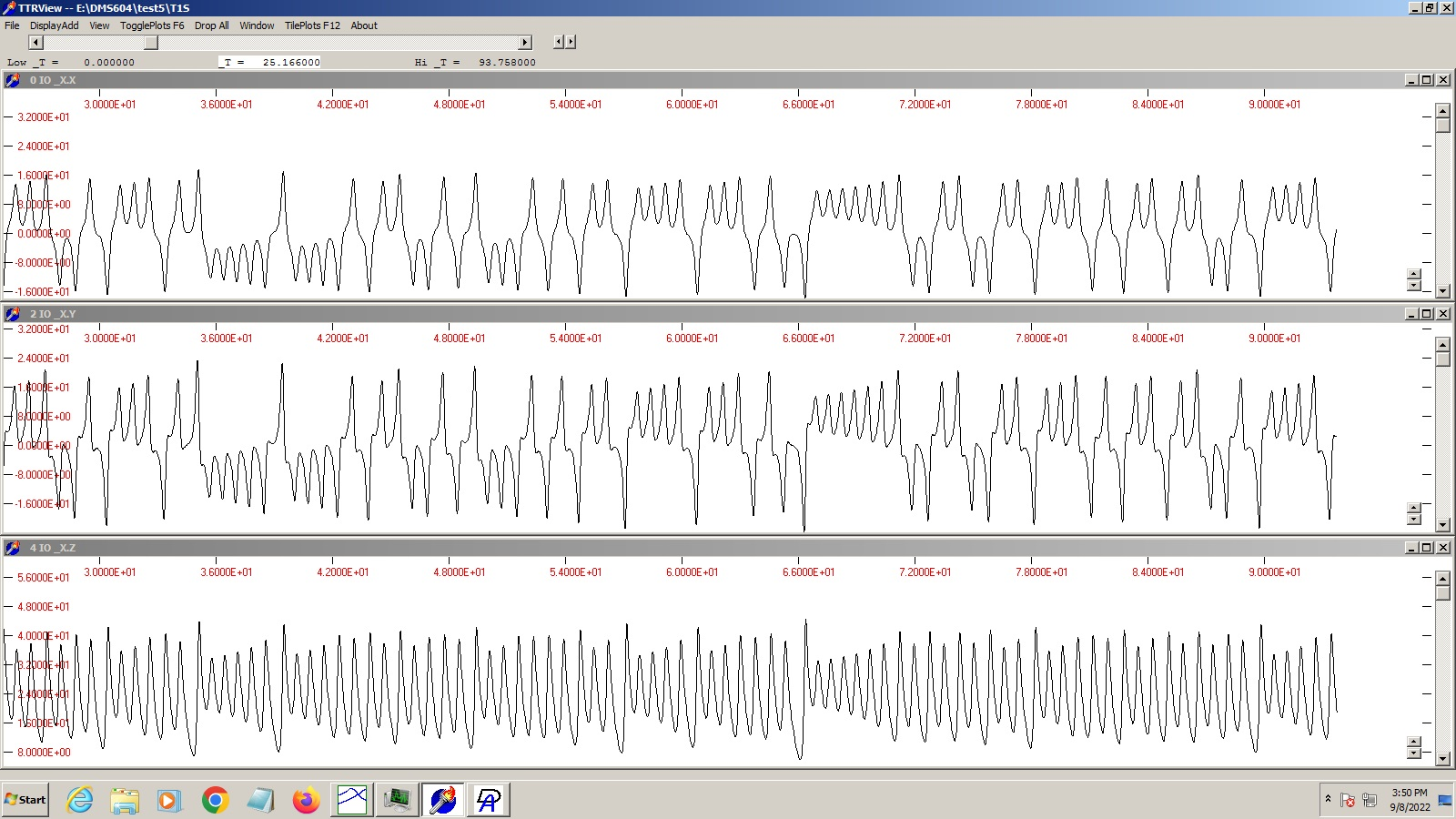### DMSolver program to compute Edward Lorenz's original "Attractor" nonlinear dynamic problem ( J. of the Atmospheric Sciences, Vol 20., pp 130-141 (1963))

This problem only requires a main module (named "SYSTEM") and is simple enough to be described by the SOSI language of DMSolver ("Single-module Only Simple Input") which the DMSolver Guide program converts for you to regular DMSolver language. The SOSI code is:

```Parameters
Sigma=10; RR=28; BB=2.666667;
Equations
Tderiv(X)=SIGMA*(Y-X);
Tderiv(Y)=-X*Z+RR*X-Y;
Tderiv(Z)=X*Y-BB*Z;
end
```

The following is not exactly the text that the SOSI converter produces because it has been made more readable and with comments, but it is mathematically equivalent:

```{ DMSolver program to compute Edward Lorenz's original "Attractor" nonlinear
dynamic problem ( J. of the Atmospheric Sciences, Vol 20., pp 130-141 (1963))}

VAR      {  global parameters must be defined to compiler}
SIGMA, RR, BB:DOUBLE;

procedure PARSET;
{lets the user adjust parameters with GUI menu; default values are supplied}
begin
_PARD(5,'Sigma parameter','','SIGMA',SIGMA,10.0);
_PARD(5,'r     parameter','','RR',RR,28.0);
_PARD(5,'b     parameter','','BB',BB,2.66667);
end;
{ Empty utility procedures are required to satisfy the linker}
procedure SETUP; begin end;  { no setup action needed }
procedure TIMESET; begin end;{ no time-varying exogenously-set values }
procedure REPORT; begin end;     { no report }
procedure ADDSET; begin end;     { not applicable }
procedure USERBPR; begin end;    {  "       "     }

{ define equation forms--simultaneous variables have special type XVR
but inside XPROC's you treat them like 8-byte reals.                       }

XPROC XDCALC(VAROT XD:XVR; VARIN X,Y:XVR);
begin
XD:=SIGMA*(Y-X);
end;

XPROC YDZDCALC(VAROT YD,ZD:XVR; VARIN X,Y,Z:XVR);
begin
YD:=-X*Z+RR*X-Y;
ZD:=X*Y-BB*Z;
end;

{ define SYSTEM module=overall block of equations and variables }

MPROC SYSTEM;
VAR
XX,XXdot,YY,YYdot,ZZ,ZZdot:XVR;  { define variables order not important}
begin    { define block of equations-order of defs not important }
XDCALC(XXdot,XX,YY);
YDZDCALC(YYdot,ZZdot,XX,YY,ZZ);
PMSINTEG(XX,XXdot);  { predefined procedure-integrate XXdot to get XX }
PMSINTEG(YY,YYdot);  { etc }
PMSINTEG(ZZ,ZZdot);
end;
```

Details and menu clicks needed to prepare and run a DMSolver problem are all described in the DMSdoc.html file. In this case I used the default parameters of Lorenz (otherwise you get different behavior) a "range" (scaling) factor of 10 for all variables (value not important in this case) and set time parameter starting at 0 and running out to several hundred units.

Starting the TTRV program with a click, and attaching the output type TTR file from the solver, and telling it to display X, Y, and Z, and picking a time range in the middle of the solution gives:Saving the TTR file to an EDTECH type DSF file, and telling EDTECH to plot Y vs X for a limited range of times gives the typical "Lorenz attractor" or "butterfly" pattern as dicussed in the book by James Gleick, Chaos: Making a New Science (1987).